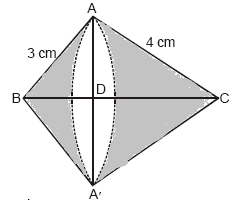# A right triangle whose sides are 3 cm and 4 cm (other than hypotenuse) is made to revolve about its hypotenuse. Find the volume and surface area of the double cone so formed. (Choose value of π as found appropriate)Given

After revolving the triangle about its hypotenuse, we get two cones having slant height as 3 cm and 4 cm each.

Hypotenuse of triangle (CD) = √(32 + 42) = 5 cm

l1 = 3 cm

l2 = 4 cm

Find out

We have to find the volume and surface area of the double cone-formed

Solution

ar(△ABC) = 1/2×AB×AC = ½×AC×CC

⇒ 4×3 = AC×5

Hence, the radius of the cone base is 12/5 cm

Now, Volume of double cone = Volume of cone 1 + Volume of cone 2

= (1/3)πr2×DC + (1/3)πr2×DB

= (1/3)πr2×(DC+DB)

= 1/3πr2×BC

On substituting the known values we get

= 30.17 cm3

Curve Surface area of double cone = CSA of cone 1 + CSA of cone 2

= πrl1 + πrl2

= πr(l1+l2)

= (22/7)(12/5)×(3+4)

=52.75 cm3

Hence, the volume and surface area of the double cone so formed is 52.75 cm3